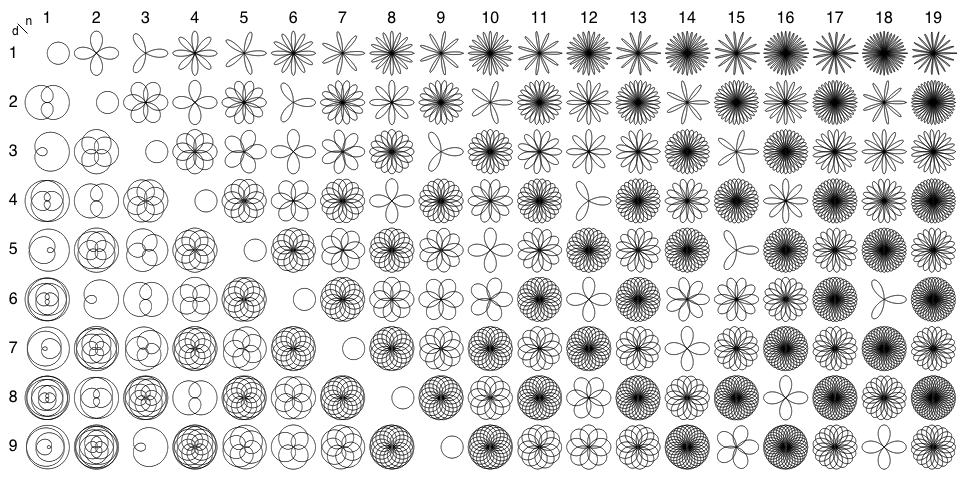# Rhodonea Curve

This is a sinusoid plotted in polar coordinates, given by r = cos(kθ) for some fixed k.

If k = p/q is rational with p and q relatively prime, then the curve has period πq when p and q are both odd, otherwise 2πq. If k is irrational, then it will have infinitely many petals.